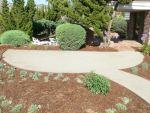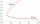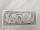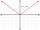# Equations

Solve following system of equations:

6(x+7)+4(y-5)=12
2(x+y)-3(-2x+4y)=-44

x =  -3
y =  2

### Step-by-step explanation:

6(x+7)+4(y-5)=12
2(x+y)-3(-2x+4y)=-44

6•(x+7)+4•(y-5)=12
2•(x+y)-3•(-2•x+4•y)=-44

6x+4y = -10
8x-10y = -44

x = -3
y = 2

Our linear equations calculator calculates it.Did you find an error or inaccuracy? Feel free to write us. Thank you!Tips to related online calculators
Do you have a system of equations and looking for calculator system of linear equations?

## Related math problems and questions:

• Two equationsSolve equations (use adding and subtracting of linear equations): -4x+11y=5 6x-11y=-5
• Eq2 equationsFor each of the following problems, determine the roots of the equation. Given the roots, sketch the graph and explain how your sketch matches the roots given and the form of the equation: g(x)=36x2-12x+5 h(x)=x2-4x+20 f(x)=4x2-24x+45 p(x)=9x2-36x+40
• Consider 2Consider the following formula: y = 3 ( x + 5 ) ( x - 2 ) Which of the following formulas is equivalent to this one? A. Y=3x2+9x-30 B. Y=x2+3x-10 C. Y=3x2+3x-10 D. Y=3x2+3x-30
• If x=4If x=4 and y=-3, what is the value of the expression x² + 2xy - 4x + 3y + y²?
• Linear systemSolve this linear system (two linear equations with two unknowns): x+y =36 19x+22y=720
• Equation 25Solve following simple equation: 3/4(x+5)=1/2(x+9)
• Non linear eqsSolve the system of non-linear equations: 3x2-3x-y=-2 -6x2-x-y=-7
• Simple equationsSolve system of equations: 5x+3y=5 5x+7y=25
• Equations - simpleSolve system of linear equations: x-2y=6 3x+2y=4
• Profit, revenue, costProfit, P(x), is the difference between revenue, R(x), and cost, C(x), so P(x) = R(x) - C(x). Which expression represents P(x), if R(x) = 3x3 + 2x - 1 and C(x) = x4 - x2 + 2x + 3?
• Linsys2Solve two equations with two unknowns: 400x+120y=147.2 350x+200y=144
• Linear systemSolve a set of two equations of two unknowns: 1.5x+1.2y=0.6 0.8x-0.2y=2
• Three points 4The line passed through three points - see table: x y -6 4 -4 3 -2 2 Write line equation in y=mx+b form
• Substitutionsolve equations by substitution: x+y= 11 y=5x-25
• Intersections 3Find the intersections of the circles x2 + y2 + 6 x - 10 y + 9 = 0 and x2 + y2 + 18 x + 4 y + 21 = 0
• Simple equation 6Solve equation with one variable: X/2+X/3+X/4=X+4
• Null pointsCalculate the roots of the equation: 3 |x +4| +3 |x +5| +2 |x +4| = 30